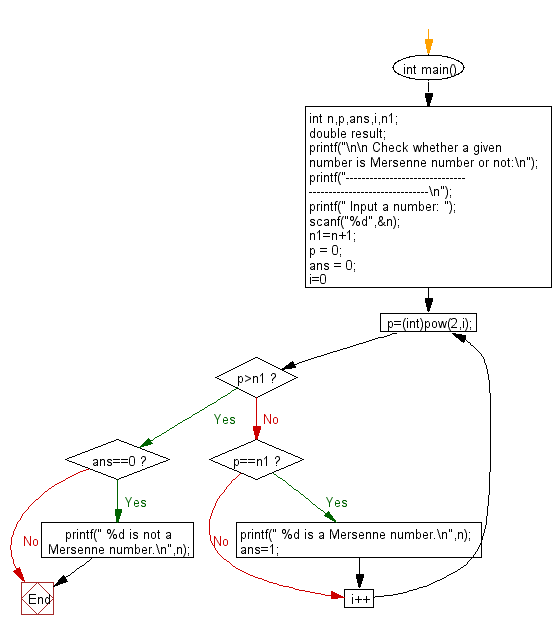﻿ C Program: Any number between 1 and n as sum of 2 cubes# C Exercises: Check if a number is Mersenne number or not

## C Numbers: Exercise-32 with Solution

Write a program in C to Check if a number is Mersenne number or not.

Test Data
Input a number: 127

Sample Solution:

C Code:

``````# include <stdio.h>
# include <stdlib.h>
# include <math.h>

int main()
{
int n,p,ans,i,n1;
double result;
printf("\n\n Check whether a given number is Mersenne number or not:\n");
printf("------------------------------------------------------------\n");
printf(" Input a number: ");
scanf("%d",&n);
n1=n+1;
p = 0;
ans = 0;
for(i=0;;i++)
{
p=(int)pow(2,i);
if(p>n1)
{
break;
}
else if(p==n1)
{
printf(" %d is a Mersenne number.\n",n);
ans=1;
}
}
if(ans==0)
{
printf(" %d is not a Mersenne number.\n",n);
}
}
```
```

Sample Output:

``` Input a number: 127
127 is a Mersenne number.
```

Pictorial Presentation:Flowchart:C Programming Code Editor:

What is the difficulty level of this exercise?

Test your Programming skills with w3resource's quiz.

﻿

## C Programming: Tips of the Day

Why do C and C++ compilers allow array lengths in function signatures when they're never enforced?

It is a quirk of the syntax for passing arrays to functions.

Actually it is not possible to pass an array in C. If you write syntax that looks like it should pass the array, what actually happens is that a pointer to the first element of the array is passed instead.

Since the pointer does not include any length information, the contents of your [] in the function formal parameter list are actually ignored.

Ref : https://bit.ly/3fhlvdH